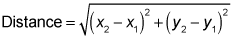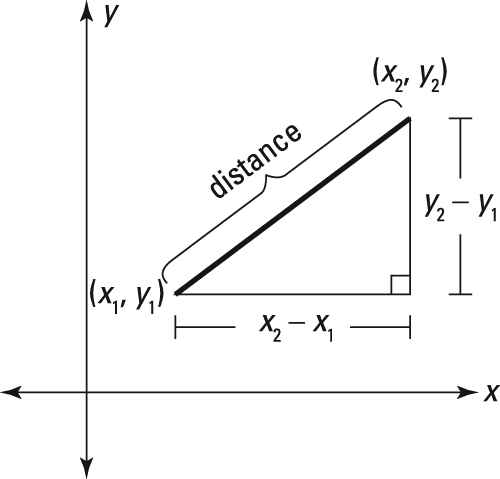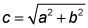##### Geometry For Dummies, 3rd EditionIf two points in the x-y coordinate system are located diagonally from each other, you can use the distance formula to find the distance between them. As you will see, this distance is also the length of a hypotenuse.

Distance formula: To calculate diagonal distances, mathematicians whipped up the distance formula, which gives the distance between two points (x1, y1) and (x2, y2):Note: Like with the slope formula, it doesn't matter which point you call (x1, y1) and which you call (x2, y2).

This figure illustrates the distance formula.The distance between two points is also the length of the hypotenuse.

As the figure shows, the distance formula is simply the Pythagorean Theorem (a2 + b2 = c2) solved for the hypotenuse:Take another look at the figure. The legs of the right triangle (a and b under the square root symbol) have lengths equal to (x2x1) and (y2y1). Remember this connection, and if you forget the distance formula, you'll be able to solve a distance problem with the Pythagorean Theorem instead.

Don't mix up the slope formula with the distance formula. You may have noticed that both formulas involve the expressions (x2 – x1) and (y2 – y1). That's because the lengths of the legs of the right triangle in the distance formula are the same as the rise and the run from the slope formula. To keep the formulas straight, just focus on the fact that slope is a ratio and distance is a hypotenuse.